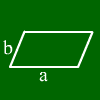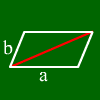# Rhomboid

In a rhomboid, these formulas hold:
a = c and b = d
alpha = gamma and beta = delta
Area = side * height on side
Further measurements are easily gotten by dividing the rhomboid into two triangles.

## What is a rhomboid?This is a rhomboid.
Typical for a rhomboid is that opposing sides are parallel. Furthermore, opposing sides have the same length, and opposing angles are equal.
In most cases, a rhomboid is uniquely defined by three values, e.g. by two sides and an angle.

## What formulas are right in a rhomboid?If you take the diagonal between opposing edges (check out the picture on the left), you can see the rhomboid as two triangles lying next to each other.
Therefore, many triangle calculation rules can give rhomboid calculation rules. For example, area A = side * height because this is twice the area formula of a triangle.

For further information, move the mouse over one of the words below, and the corresponding part is marked on the rhomboid.Side a Side b
Angle alpha Angle beta
Diagonal e Diagonal f
Height on a Height on b
Area

## Calculating a rhomboid

Mathepower can do all kinds of area calculations. Calculating the area of a rhomboid is also no problem. Just enter side, height, anges or area of the rhomboid.